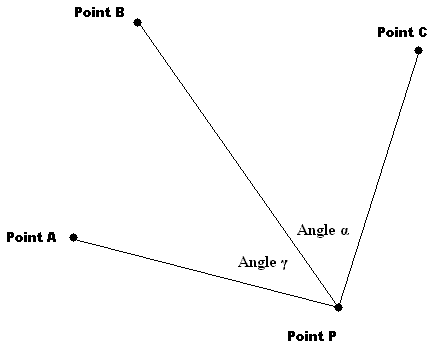## 3-Point Resection Solver

### Using Tienstra's Method

#### by Mesa Mikemike@mesamike.org

Calculate the coordinates of Point P given three known reference points and the observed angles between them from Point P.
This is an old surveyor's technique, not used much any more these days.
If you want to see how it's done, use your browser's "view source" function.

NOTE: The results are indeterminate (that means "wildly inaccurate") if all four points lie on (or sufficiently close to) a circle or the 3 known reference points lie on a straight line. This is an unavoidable limitation of Tienstra's method.

A FURTHER CAVEAT: Professor Ken Snelgrove of Memorial University (St. John's, Newfoundland & Labrador) writes to inform us of an additional limitation of Tienstra's Method that has caused some unexpected answers for his students when using this solver. The problem is that unless point P lies within the triangle defined by points A, B and C, there exist 2 points P that will satisfy the given inputs. Tienstra's Method (and this solver) will compute only one of them, possibly the wrong one. The method computes the wrong point when the angle subtended by points A, B and C is greater than 180 degrees. For example, if in the diagram below Point B were on the Point P side of the line connecting Points A and C.

ALSO NOTE: This method is based on simple plane trigonometry and does not provide a geodetic solution (that is, it doesn't take into account the curvature of the Earth). However, for geocaching purposes, the results are "close enough" if all the points in question are within, say, 50 miles or so of each other.X Y
(easting) (northing)
Point A
Point B
Point C
Angle α (degrees)
Angle γ (degrees)
Point P

### Other Results of Interest

 distance AB distance AC distance BC distance AP distance BP distance CP angle BAC degrees angle ABC degrees angle ACB degrees